###### How to Solve Word Problems Programmatically

Chapter

2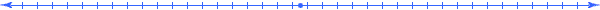Chapter 2.  Extending Unit Analysis

 2.1 Unit as a Meaning 2.2 Unit Identity 2.3 Unit Conversion 2.4 Proportionality 2.5 Constant of Proportionality 2.6 Cancellation Rule 2.7 Constant Rate 2.8 Unit Chains 2.9 The Domino Strategy 2.10 Combining Proportional Quantities 2.11 Activities and Explorations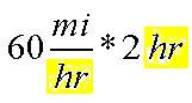2.1

#### Unit as a Meaning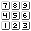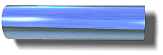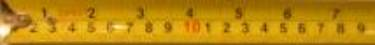This instance of a metal bar has a length dimension whose quantity corresponds to either 3 in or 7.62 cm.

A unit specifies an amount of a given dimension.  For example, there exists a metal bar stored in France that the metric system used to represent the standard size for a meter of length.  Units provide the basic building blocks of measurement.  The above measuring tape has two unit scales on it, one for inches (in) and one for centimeters (cm).  We use multiples of a unit to measure other instances of an object.  The 3-inch bar measured above is 3 times as large as a metal bar that corresponds to one inch (a unit inch).

 In general, a unit of a given dimension D of a given thing T represents a quantity 1 of the dimension D for a specific occurrence of the thing T.  Each other quantity q corresponds to an occurrence of that thing that is q times as much.    If we measure the same occurrence of  a thing using two different units then the resulting unified quantities are equal. q [u~D(T)]   3 [in~Length(Bar)] = 7.62 [cm~Length(Bar)]   3 in = 7.62 cm

 1 in = 2.54 cm 1 [in~Dimension(Thing)] = 2.54 [cm~ Dimension(Thing)]    16 oz = 1 lb 16 [oz~Dimension(Thing)] = 1 [lb~ Dimension(Thing)] Equal Unified Quantities

When we abbreviate and only mention units in unified quantities and equations, we assume that the units measure their corresponding default dimensions of a common thing.

2.2

#### Unit IdentityWe frequently encounter equations used to express unit conversions such as "3 ft = 1 yd".   If we divide a unit conversion equation like "3 ft =1 yd" with the left-hand-side expression "3 ft" we obtain the equation "1 = 1 yd / 3 ft".   Similarly, if we divide that same unit conversion equation "3 ft = 1 yd" with the right-hand-side expression "1 yd" we obtain the equation "3 ft / 1 yd = 1".   These new statements imply that both the expressions "3 ft / 1 yd" and its inverse "1 yd / 3 ft" are unit identity expressions that we can use as factors to multiply other unified quantities without changing the actual amount of that dimension.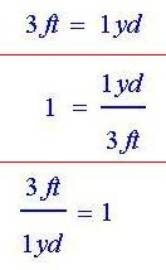We can also express "3 ft / 1 yd" as "3 ft/yd" (read "3 feet per yard") and "1 yd / 3 ft" as ".33 yd/ft".  Each of these four expressions provides examples of unit identities.  Some unit identifies result from approximations such as "1 in ≈ 2.54 cm" or the result of round off error such as "1/3=.33".  In these situations, we will still use the equal sign with an understanding that it really means "nearly equal to".

 1 in = 2.54 cm 2.54 cm/in .394 in/cm 1 min = 60 s 60 s/min .0167 min/s 1 gal = 4 pt 1 lb = 16 oz 1 kg = 1000 g

Fill in the unit identity factors

Go ahead and fill in the blanks.  See Unit Conversions Appendix for more examples.

The web page http://matti.usu.edu/nlvm/nav/frames_asid_272_g_3_t_4.html contains a simple applet that helps you practice using conversion factors and their inverses.

2.3

#### Unit ConversionWhen converting from one unit to another, we use the unit identity that has the same unit in the denominator as the unit of the unified quantity that it multiplies.  So if we want to convert the unified quantity "3 in" into centimeters, we would multiply by the unit identity "2.54 cm/in" which results in multiplying the quantity 3 by 2.54.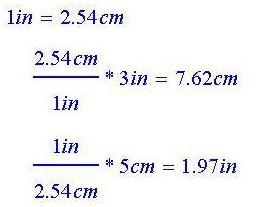When do we multiply?       When do we divide?

On the other hand, if we want to convert "5 cm" to inches, we would multiply by the unit identity "(1/2.54) in/cm" which results in dividing the quantity 5 by 2.54.   This idea is widely used in the physical sciences like physics and chemistry to solve certain word problems, but we will now extend the idea of unit conversions and cancellation to many other fields such as accounting, finance, architecture, and home economics to name a few.

2.4

#### Proportionality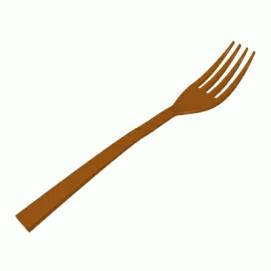3 [in~Length(Fork)] 6 [in~Length(Fork)] 9 [in~Length(Fork)] .2 [in~Width(Fork)] .4 [in~Width(Fork)] .6 [in~Width(Fork)] 3 / .2 = 15 6 / .4 = 15 9 / .6 = 15

If the size of a thing grows proportionally, then each length dimension increases by the same multiple as the other dimensions.  If we double the width dimension, then the length dimension would double.  If we triple the width dimension, the length dimension would triple; and other proportional dimensions like "gap between tongues" would triple.    In general two scaled dimensions are proportional, if the increase of one scaled dimension by a multiple causes the other scaled dimension to increase by the same multiple.

2.5

#### Constant of Proportionality3 [in~Length(Fork)] = 1/5 [in~Width(Fork)]   15 [in~Length(Fork)] / [in~Width(Fork)]   (1/15) [in~Width(Fork)] / [in~Length(Fork)] One unified equation from a proportional relationship   Constants of Proportionality

Unified Math™ uses the new concepts of meaning to extend traditional unit analysis used extensively in physical sciences.  Just like "3=1" did not make any sense until we added units (3 ft = 1 yd), the equation "3=1/5" above does not make sense unless we added the complete meaning and interpreted the equality as stating that these 2 values occurred on the same instance of a thing.  So just like 3 feet and 1 yard occur on the same instance of a thing, we extend this definition of "equality" to any two unified quantities in a system of things so long as the things increase proportionally.  In such equations, we interpret the equal sign (=) as "corresponds to".

Thus we express the relationship between two proportional dimensions as a unified equation similar to the way that we express the relationship between convertible units.  On dividing the equation by one side, we obtain a constant of proportionality.  Notice that for any instance of the proportional forks, the fraction of the quantities of these two dimensions equals the constant of proportionality.

We use constants of proportionality similar to unit identities.  They convert dimensions as well as units.  For example, if we have a fork that is proportional to these other forks but has a width of .5 inches, then we calculate the length by multiplying it by the constant of proportionality having width in denominator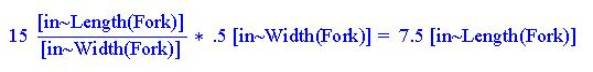Notice the key role that proportionality plays to allows us to extend the idea of "equality" to unified quantities.  Consider some unified equations and their corresponding constants of proportionality for the thing "Circle".

 2 [ft~Diameter(Circle)] = 1 [ft~Radius(Circle)] 2 [ft~Diameter(Circle)] / [ft~Radius(Circle)]   π [m~Circumference(Circle)] = 1 [m~Diameter(Circle)]  π [m~Circumference(Circle)] / [m~Diameter(Circle)]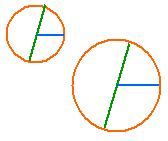Notice that the resulting ratios are not unit identities; they are constants of proportionality.  On the other hand, a unit identity can be considered as a special case of a constant of proportionality where the scaled dimensions only differ by their units as illustrated below:

 2.54 [cm~Length(Board)] = 1 [in~Length(Board)] 2.54 [cm~Length(Board)] / [in~Length(Board)]   4 [cups~Volume(Jar)] = 1 [qt~Volume(Jar)]  4 [cups~Volume(Jar)] / [qt~Volume(Jar)] Unit Identities are Constants of Proportionality

2.6

#### Cancellation Rule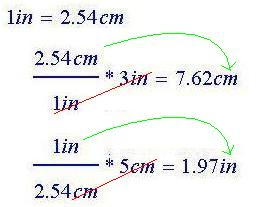The inch (in) unit cancels out leaving only "cm".       The centimeter (cm) unit cancels leaving "in".

We can think of this process of multiplying by a unit identity or constant of proportionality as a process of canceling units.  When converting the unified quantity "3 in" to centimeters, the inch (in) unit cancels.  When converting the unified quantity "5 cm" to inches the centimeter (cm) unit cancels.

 When multiplying two unified quantities, we can cancel matching units if one unified quantity is a constant of proportionality with a denominator meaning that matches the meaning of the other unified quantity. Cancellation Rule

Here are some examples of constants of proportionality involved in the cancellation rule: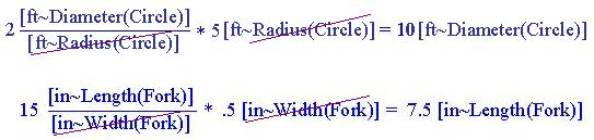2.7

#### Constant RateConsider the speed of a car as an example of rate.  This rate is not constant when the car accelerates; but after it reaches a cruising speed (a constant speed) then we have a constant rate.   At a constant rate of 50 miles per hour (mi/hr), for example, if we change the time of the trip from 2 hr to 5 hr (a difference of 3 hr) then the distance will change from 100 mi (obtained from 50 mi/hr * 2 hr) to 250 mi (obtained from 50 mi/hr * 5 hr), a difference of 150 mi.  Notice that the constant rate of 50 mi/hr times the difference of 3 hr does indeed give us the expected 150 mi. [hr~ Time(Trip)]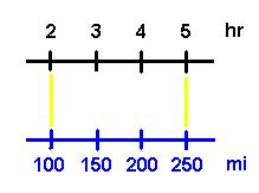[mi~ Length(Trip)]

 [in~ Length(Thing)]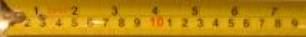[cm~Length(Thing)] Notice how the above diagram of the scaled dimensions [hr~Time(Trip)] and [mi~Length(Trip)] parallel each other in the same manner as centimeters and inches line up on a ruler.

 In Unified Math® a rate expresses how a unified quantity change as another unified quantity changes when the thing changes.  In later chapters, we will present the rate as a (derivative) function.  For now, we think of a constant rate as the constant of proportionality between the changes in two proportional unified quantities.   By the cancellation rule,  if one quantity changes by a certain amount, then the other quantity changes by that amount times the constant of proportionality. 2 m/s .5 s/m 60 mi/hr 7.95 dol/box 22 mi/gal 50 people/show 2.54 cm/in 3 ft/yd Examples of Constant Rates

In general, a constant rate can multiply unified quantities that have meanings that match the meaning in the denominator of the rate and the cancellation rule apply.

 2 m/s *10 s = 20 m .5 s/m *20 m = 10 s 60 mi/hr *3 hr = 180 mi 7.95 dol/box *2 box = 15.90 dol 22 mi/gal *3 gal = 66 mi 50 people/show *4 show = 200 people 2.54 cm/in *1 in = 2.54 cm 3 ft/yd *200 yd = 600 ft Multiplying constant rates with abbreviated unified quantities

2.8

#### Unit Chains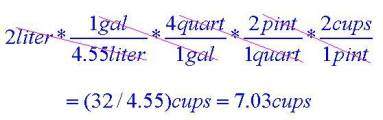Unit Chain

To construct a unit chain, begin with a unified quantity (like "2 liter" in this example) and repeatedly multiply by constant rates (including unit identities and constants of proportionality) where meaning in the numerator of the resulting unified quantity matches the denominator of the next unified quantity being multiplied.  The first multiplication in this example matches the unit "liter" and the last multiplication matches the unit "pint".

2.9

#### The Domino StrategyWord Problem Solution Using Units Analysis What is the mass (in kilograms) of a person who weighs 180 pounds (lb).  (Hint:  1 lb = .453 kg) x kg = (.453 kg/ lb) * 180 lb = 81.5 kg

If a word problems involves only multiplication and division operators, one of the most effective solution strategy, which we will call the domino strategy, creates a chain of factors starting with a unified quantity having the desired meaning (highlighted in yellow) in the numerator of its meaning expression and continues to multiply by unified quantities that again has a numerator meaning matching the previous denominator meaning.   In such cases the factors usually involve unit conversions and constant rates.  In the example above, we want to find kilograms (kg) so we start with the unified quantity .453 kg/lb which has the unit kg in the numerator.  Then we use the unit "lb" in the numerator to select the next factor 180 lb.  The first factor in this example is a unit conversion.  In this next example, the first factor used is a constant of proportionality.

 Word Problem Solution Using Units Analysis If the car can get 30 miles per gallon (mi/gal),  what distance (in miles) can the car travel before it runs out of 15 gallons? x mi = (30 mi/gal) * 15 gal = 450 mi

Here we desire the unit miles to begin the domino strategy.  We again can multiply by 15 gal to cancel out the unit "gal".  Go ahead and solve the following problems and check the answers below.

 Word Problem Solution Using Units Analysis How many hours will it take a truck traveling 40 miles per hour to go 160 miles? With a price of 2 dollars per quart, how many quarts can a person buy with 30 dollars? How much money do we need to purchase 3 liters (L) of chemicals if 2 gallons (gal) of chemicals cost 4 dollars (dol)?  (Hint:  1 gal = 3.78 L) How many seconds in a day? How fast is a car going in miles per hour if the odometer reads 90 kilometers per hour?  (Hint: 1.609 m = 1 mi)

Here are some domino solutions for the above problems.

 Word Problem Solution Using Units Analysis What is the mass (in kilograms) of a person who weighs 180 pounds (lb).  (Hint:  1 lb = .453 kg) x kg = (.453 kg/ lb) * 180 lb = 81.5 kg If the car can get 30 miles per gallon (mi/gal),  what distance (in miles) can the car travel before it runs out of 15 gallons? x mi = (30 mi/gal) * 15 gal = 450 mi How many hours will it take a truck traveling 40 miles per hour to go 160 miles? x hr = (1 hr / 40 mi) * 160 mi = 4 hr With a price of 2 dollars per quart, how many quarts can a person buy with 30 dollars? x qt = (1 qt / 2 dol) * 30 dol = 15 qt How much money do we need to purchase 3 liters (L) of chemicals if 2 gallons (gal) of chemicals cost 4 dollars (dol)?  (Hint:  1 gal = 3.78 L) x dol = (4 dol/2 gal) * (1 gal/3.78 L) * 3 L = 22.68 dol How many seconds in a day? x s = (60 s/min)*(60 min/hr)*(24 hr/d)* 1 d = 86400 s How fast is a car going in miles per hour if the odometer reads 90 kilometers per hour?  (Hint: 1.609 m = 1 mi) x mi/hr = (1 mi / 1.609 km)* (90 km/hr) = 56 mi/hr

2.10

#### Combining Proportional QuantitiesEnglish Phrase Expression Meaning 4 boys per 12 students 4/12 boys / student 10 meters every 2 seconds 10/2 meters / second 30 pools in 50 homes sampled 30/50 pools / home 20 percent of the cars have CD Players 20/100 CD players / car 1 out of 3 investments were loans 1/3 loans / investment

Sometimes, we need to combine two or more numbers or variables into one quantity in order to identify the meaning.  This typically occurs in describing the ratio associated with a constant of proportionality.   Notice that the word percent implies per 100, a number that would appear in the denominator of the resulting expression.  We will illustrate this in the next word problem.

2.11

#### Activities and ExplorationsActivities:

 Practice Using Unit Identities http://matti.usu.edu/nlvm/nav/frames_asid_272_g_3_t_4.html   Play “Unit Chain Gang” game Start with a unified quantity and build a chain by multiplying with constant rates.  Each team has a short amount of time to suggest a new constant rate factor that matches its denominator meaning with the numerator meaning of the growing chain.  The constant rates must be different during a round of play.  Optionally, you can play this game as individuals and drop out each player who cannot suggest a new constant rate factor within the time frame.  See Unit Conversions Appendix for ideas.   Play "Word Problem Torpedo" game Each team takes turns creating a constant-rate word problem (the torpedo) and fires it at the other team.  The other team then attempts to solve.   Play "Recipe Swapping" game Each team creates a (reasonable) recipe (either from scratch or from ideas in recipe books) that contains at most ten items.  The teams swap recipes and race to convert the recipes to the metric system.  Each correct conversion gives that team a point.  Each incorrect conversion gives the other team that point.

Explorations:

 Cancellation of Units Explained (Purplemath)   Units Analysis   Examples of Canceling Units http://www3.cerritos.edu/earth-science/tutor/math/units_of_measurement.htm http://math.whatcom.ctc.edu/stuhelp/faq/elemalg/units1.htm http://www.wwnorton.com/chemistry/concepts/chapter1/ch1_4.htm   Advanced Example http://www.theinformant.com/problem.html   A Grid Tool for Units Analysis http://www.webs1.uidaho.edu/pdm/tools/units.htm   Dr. Math on Dimensional Analysis (Unit Analysis) http://mathforum.org/library/drmath/view/56728.html (Note his comment that it is missing in many school curriculums)   Explore Unit Conversions Unit Conversions Appendix

Exercises:

 Do Related Problems In Your Textbook   Using Meaning Table Use the domino strategy to solve word problems in

Copyright © 2004  Dr. Ranel E. Erickson